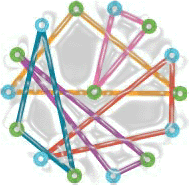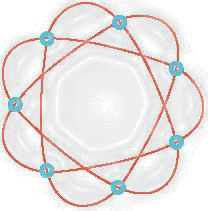# Projective spaceThis is a generator-only picture of the smallest projective space. This geometry has 15 points, 35 lines and 15 planes. Every line contains 3 points, there are 7 lines through every point, all planes are Fano planes, two distinct points are contained in exactly one line and any three points that do not form a line are contained in exactly one plane. In the diagram the 15 points are the 15 blue and green circles and the lines are generated by the 5 lines/triangles by rotating the diagram 7 times 360/7 degrees at a time around its center.

 It is a little bit harder to see the planes except for one which sits right in the middle of the diagram. Its points are the blue circles and its lines are generated by the red line. Another remarkable fact about this picture is that the five generator lines partition the point set of the space. This means that they form a spread of lines. Furthermore, the 6 images of this spread are also spreads and all 7 spreads partition the line set of the space. A partition like this is called a packing.